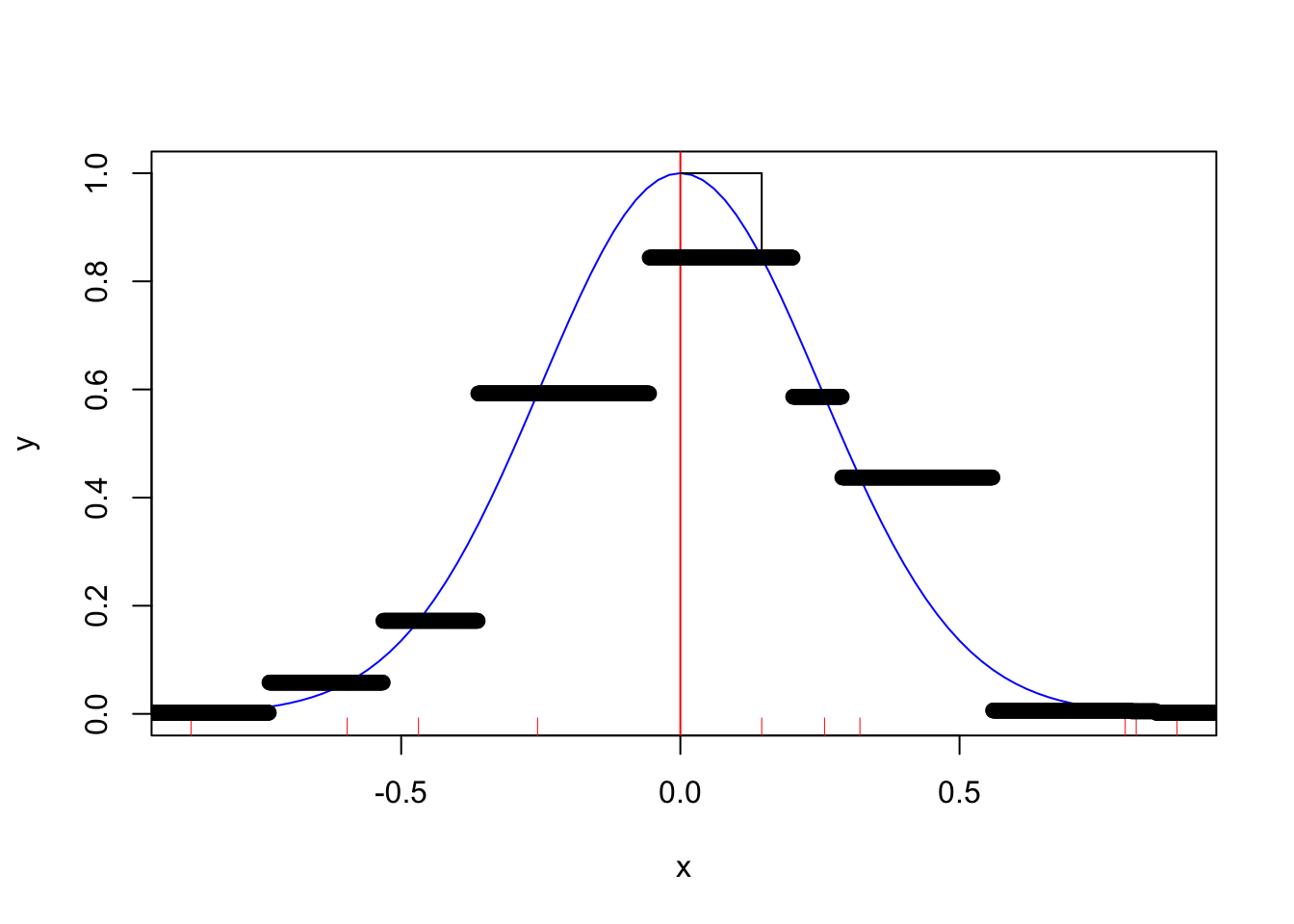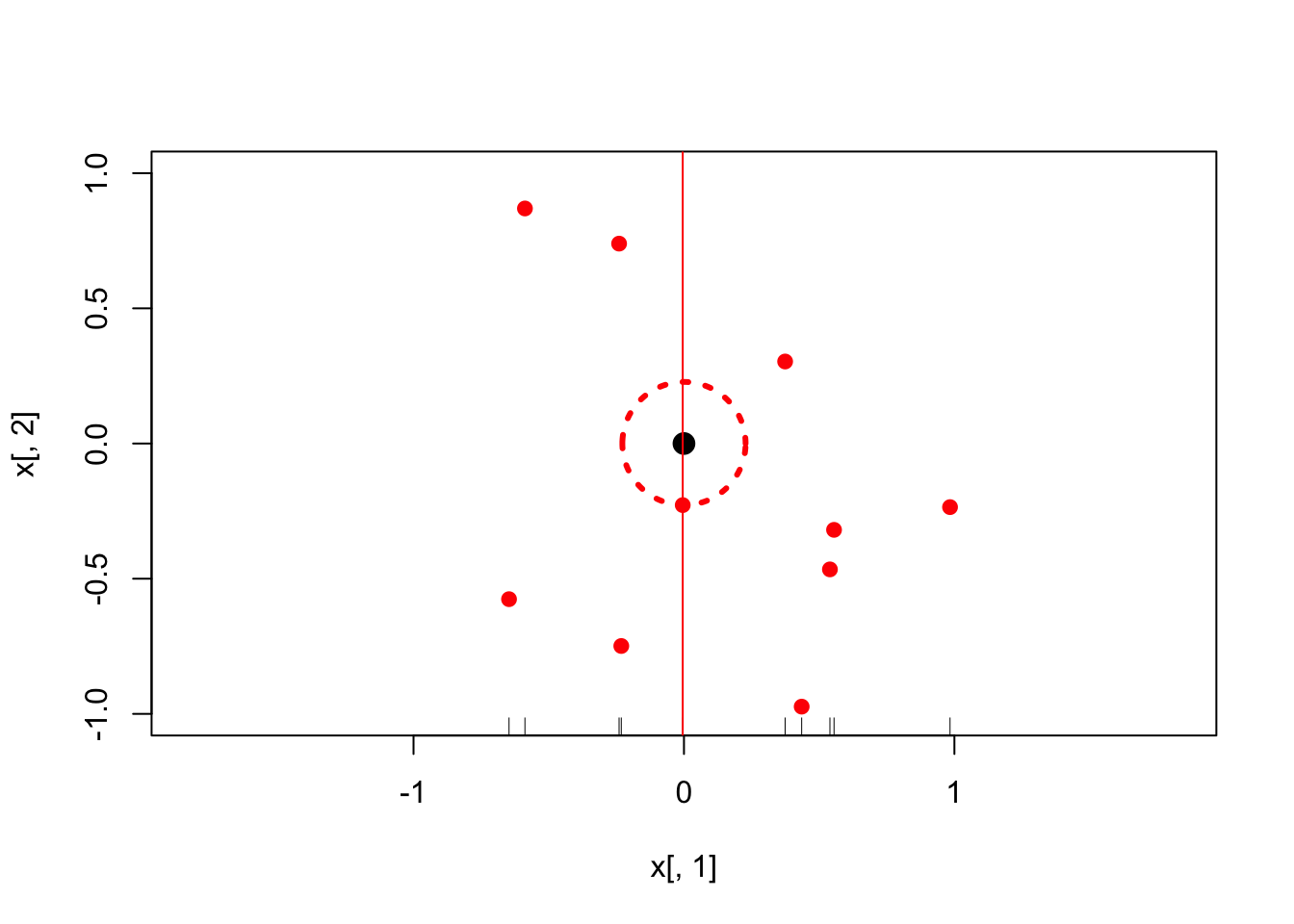#### Curse of Dimensionality

Examples stats-curse-dimensionality.utf8

## Example Curse of Dimensionality

We want to study the distance between a target point and it’s nearest neighbor as the dimension increases.

We first we study p=1 dimension

set.seed(1)

Generate 10 x_i uniformly on [−1, 1]

p <- 1
n <- 10
x <- runif(n, -1, 1)

The True noiseless relationship is $$y = e^{-8||x||^2}$$

y <- exp(-8*x^2)
plot(x, y, ylim = c(0,1), pch = 19, col = "red")
abline(v = 0, col = "red")
curve(exp(-8*x^2), from = -1, to = 1, col = "blue", add = TRUE)
rug(x, col = "red")

#Calculate the nearest neighbor to target 0
hat_y <- y[which.min(abs(x))]
min_x <- x[which.min(abs(x))]

lines(c(min_x, min_x), c(hat_y, 1))
lines(c(0, min_x), c(1, 1))

# Let's give the kNN estimate for k=1

x_seq    <- seq(-1, 1, by = .001)
seq_mat  <- matrix(rep(x_seq, each = length(x)), ncol = length(x), byrow = TRUE)
x_mat    <- matrix(rep(x, each = length(x_seq)), nrow = length(x_seq))
dist_mat <- (seq_mat - x_mat)^2
y_seq    <- y[apply(dist_mat, 1, which.min)]
points(x_seq, y_seq)Next we study p=2 dimension2

Generate 10 x_i uniformly on [−1, 1]^2

p <- 2
n <- 10
x <- matrix(runif(n*p, -1, 1), nrow = n, ncol = p)

The True noiseless relationship is $$y = e^{-8||x||^2}$$

nrm_x <- apply(x^2, 1, sum)
y     <- exp(-8*nrm_x^2)
plot(x[, 1], x[, 2], xlim = c(-1,1), ylim = c(-1,1), asp = 1, pch = 19, col = "red")
points(0, 0, pch = 19, cex = 1.5)

# Calculate the nearest neighbor to target (0,0)

hat_y <- y[which.min(nrm_x)]
min_x <- x[which.min(nrm_x), ]
dist_2 <- sqrt(nrm_x[which.min(nrm_x)])

theta  <- seq(0, 2 * pi, length = 200)
lines(x = radius * cos(theta), y = radius * sin(theta), col = "red", lty = 3, lwd = 3)

# Consider projecting the data onto the first dimension, the nearest neighbor is much closer

rug(x[, 1])
one_dim_min_x <- x[which.min(abs(x))]
abline(v = one_dim_min_x, col = "red")dist_1 <- abs(one_dim_min_x)

Let’s calculate 1000 simulations in each of 1 and 2 dimensions when n = 10

sims <- 1000
dist_1 <- rep(NA, sims)
dist_2 <- rep(NA, sims)

p1 <- 1
p2 <- 2
n  <- 10

for (i in 1:sims) {
x1 <- matrix(runif(n*p1, -1, 1), nrow = n, ncol = p1)
x2 <- matrix(runif(n*p2, -1, 1), nrow = n, ncol = p2)

nrm_x1 <- apply(x1^2, 1, sum)
nrm_x2 <- apply(x2^2, 1, sum)

dist_1[i] <- sqrt(nrm_x1[which.min(nrm_x1)])
dist_2[i] <- sqrt(nrm_x2[which.min(nrm_x2)])
}

mean(dist_1)
##  0.08862825
mean(dist_2)
##  0.309566

Let’s calculate 1000 simulations in each of 1 and 2 dimensions when n = 1000

sims <- 1000
dist_1 <- rep(NA, sims)
dist_2 <- rep(NA, sims)

p1 <- 1
p2 <- 2
n  <- 1000

for (i in 1:sims) {
x1 <- matrix(runif(n*p1, -1, 1), nrow = n, ncol = p1)
x2 <- matrix(runif(n*p2, -1, 1), nrow = n, ncol = p2)

nrm_x1 <- apply(x1^2, 1, sum)
nrm_x2 <- apply(x2^2, 1, sum)

dist_1[i] <- sqrt(nrm_x1[which.min(nrm_x1)])
dist_2[i] <- sqrt(nrm_x2[which.min(nrm_x2)])
}

mean(dist_1)
##  0.0009956784
mean(dist_2)
##  0.03176299

## Section

Txt txt txt txt txt txt txt.